# How to Convert Transmittance to Absorbance?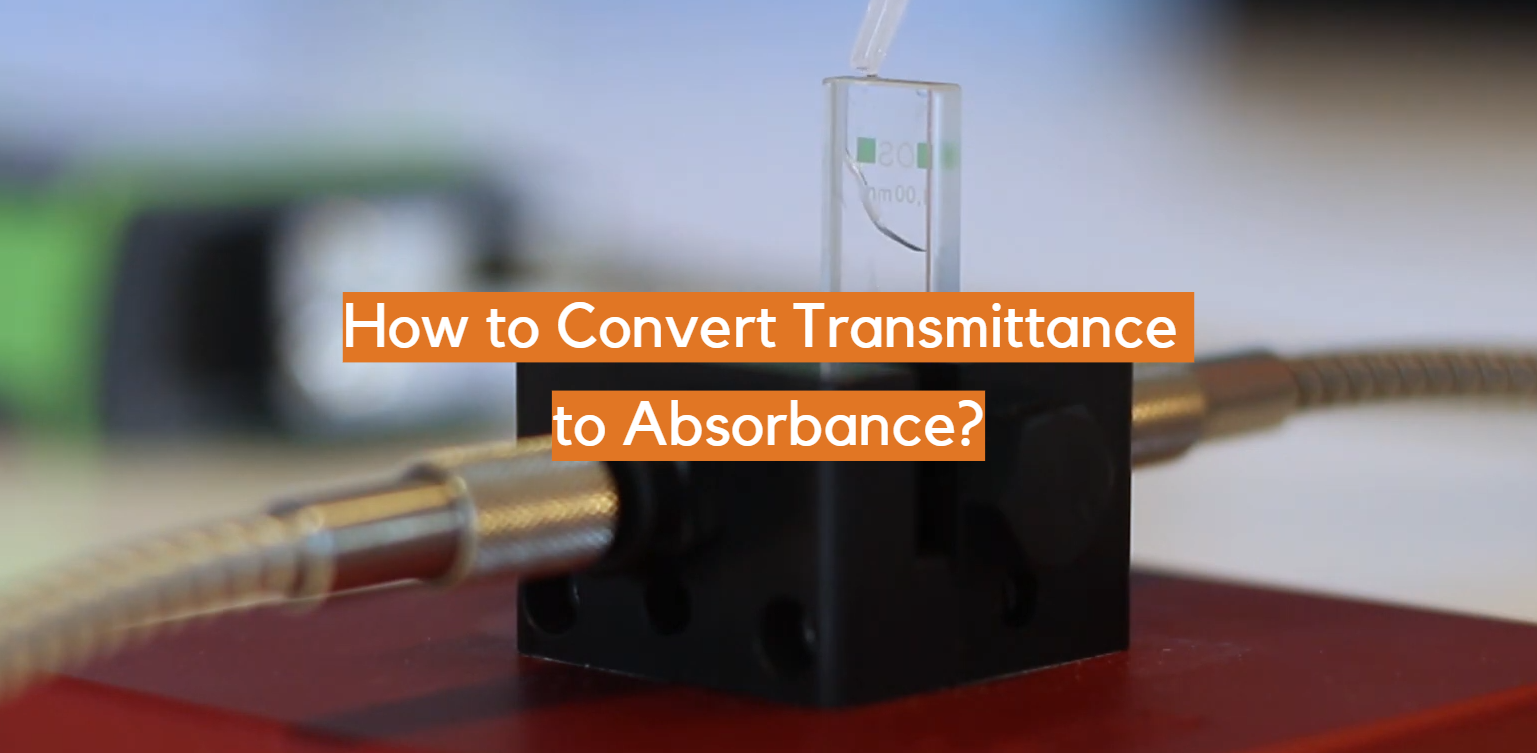When you find yourself needing to measure the amount of light that passes through a certain material, one way to do so is with transmittance. Transmittance is simply the proportion of light that passes through an object or medium in comparison with what would pass without any obstacles at all. This can be expressed as a percentage and it’s used in industries such as manufacturing, electronics, space science and more. But sometimes you need to use absorbance instead – which measures how much light was actually absorbed by the material rather than just passing through it. If this sounds confusing don’t worry! In today’s blog post we guide you through how to convert from transmittance to absorbance – providing simple formulas and practical tips for understanding this difference plus much more.

## How to Convert Transmittance to Absorbance

### Calculation Method

To convert transmittance to absorbance, the equation A = log10(T/100) must be used, where A is absorbance and T is transmittance. To use this equation, simply plug in the known values of transmittance and solve for absorbance.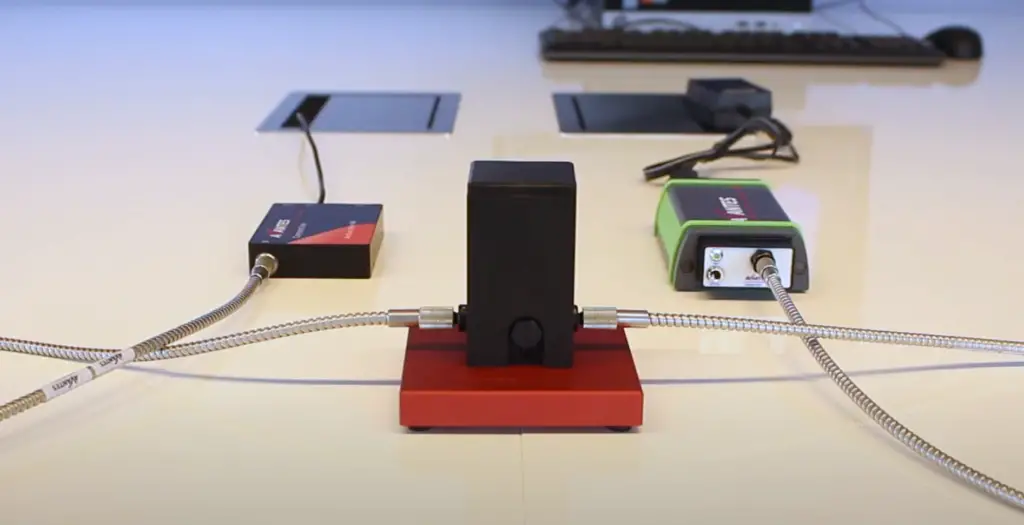For example, if a given sample has a transmittance of 50%, then its corresponding absorbance can be calculated as follows:

A = log10 (50/100) A = – 0.3010

Therefore, the sample with a transmittance of 50% has an absorbance of -0.3010. It is important to note that this calculation assumes that all light passing through the sample is measured at a single wavelength. 

In some instances, it may be necessary to convert multiple values of transmittance to absorbance. In these cases, an absorbance-transmittance table can be used as reference. This table will contain various values of transmittance and their corresponding absorbances. For example, if the value of transmittance is 75%, then its corresponding absorbance would be found in the table to be -0.1761. When using this method, it is important that all data points are collected from the same light source and under the same conditions (e.g., temperature, pressure, etc.). Additionally, any losses due to absorption or reflections should also be taken into account when performing the calculations.

### Nomogram Method

Another method for converting transmittance to absorbance is by using a nomogram. A nomogram is a graphical representation of an equation, which can be used to quickly calculate the values of various parameters. To use a nomogram for this conversion, one first must locate the transmittance value on the x-axis and then move along a straight line until it intersects with the y-axis.

This intersection indicates the corresponding absorbance value. For instance, if the value of transmittance is 40%, then its corresponding absorbance would be found in the nomogram to be -0.3993. It should also be noted that many modern instruments are now equipped with built-in algorithms which can directly convert transmittance to absorbance. It is important to note that when using either of these methods, all readings should be taken at a single wavelength and in the same conditions (e.g., temperature, pressure, etc.). Additionally, any losses due to absorption or reflections should also be taken into account when performing the calculations.

By carefully following these steps, it is possible to accurately convert transmittance values into absorbance values.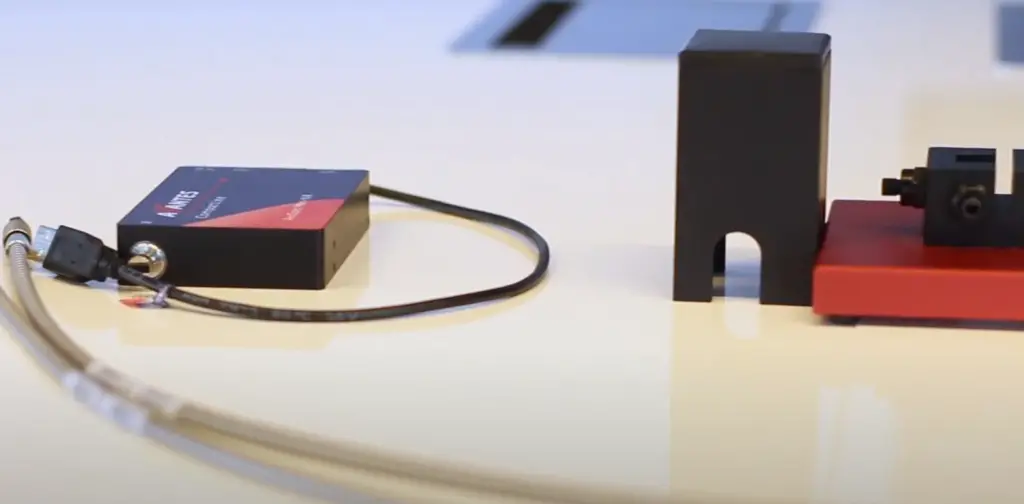### Experimental Method

The conversion of transmittance to absorbance can be done experimentally. The following steps should be followed in this process:

1. Measure the intensity of light passing through a sample using an instrument like a spectrophotometer. This is known as the transmitted intensity, I_t.
2. Calculate the total incident light by measuring its intensity before it passes through the sample. This is known as the incident intensity, I_o.
3. Divide the transmitted intensity by the incident intensity and multiply it by 100 to obtain transmittance (T).
4. To find absorbance (A), use the equation A=-log10(T/100) or A=-log10(I_t/I_o).
5. Convert absorbance to % transmittance by using the equation T = 10^(-A) * 100.

By following these steps, one can easily convert transmittance to absorbance. Additionally, it is important to make sure that the light used in this experiment is monochromatic (i.e., of a single wavelength or color). 

## Convert Transmittance to Absorbance in Excel

To convert transmittance to absorbance in Excel, you will need to use a formula. The formula is: A=-log10(T/100). Here, “A” stands for absorbance and “T” stands for transmittance (measured as a percentage).

### Example: Convert 40% transmittance to absorbance.

The value of T would be 40 and the formula for calculating absorbance becomes A=-log10(40/100) = 0.398. Therefore, the absorbance of 40% transmittance is 0.398 or -0.398 log units.

When entering this into an Excel spreadsheet, you would enter: =-LOG10(40/100). This will give you the absorbance value of 0.398 or -0.398 log units.

This same formula can be used to convert transmittance to absorbance for any transmittance percentage, simply by inputting the correct values into the equation. Keep in mind that when using this method, you should always use two decimal places for accuracy and enter all values as percentages rather than fractions.

By following these steps, you can easily calculate absorbance from transmittance in Excel. Doing so can help you quickly determine how much light is being absorbed by a sample without having to use more advanced scientific instruments or laboratory techniques.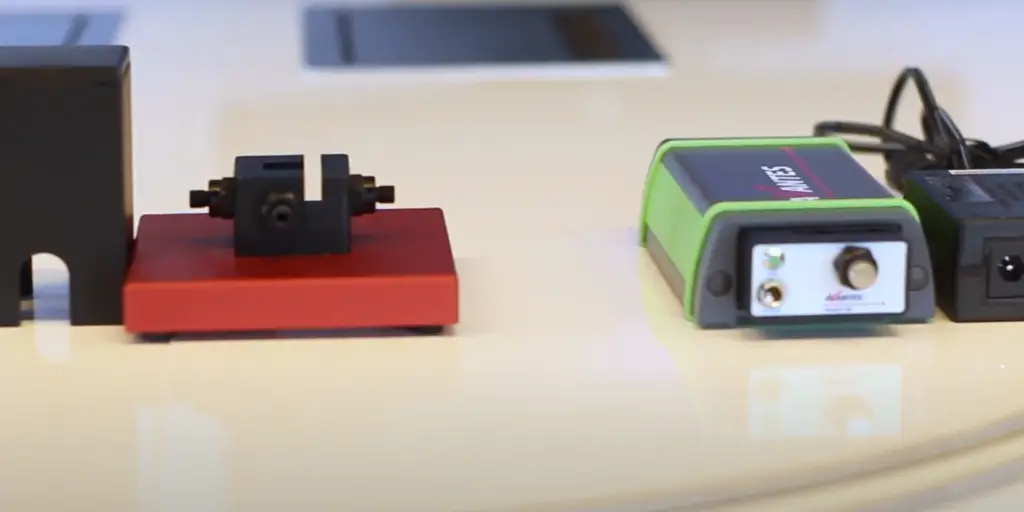## Beer-Lambert Law

Beer-Lambert Law, also known as Beer’s Law or Lambert-Beer Law, is used to convert transmittance to absorbance. The Beer-Lambert law states that the absorbance of light (A) through a sample is directly proportional to the concentration of the absorbing material (C) in a sample and the path length (b) that light travels through it. Mathematically, it can be expressed as A = ε x b x C. Here, ε represents the molar absorptivity coefficient which varies for different materials and wavelengths of light.

To convert transmittance (%) to absorbance, first calculate the transmission ratio by dividing the transmitted intensity by incident intensity (T/I). Then take the negative logarithm of the ratio to obtain absorbance. Mathematically, it can be expressed as A = -log (T/I). 

For example, if the transmittance of a sample is 85%, then the transmission ratio is 0.85 and hence its absorbance will be A = -log (0.85) = 0.078. The absorbance can further be used in Beer-Lambert law to estimate sample concentration or molar absorptivity coefficient depending upon the application at hand.

Thus, Beer-Lambert Law helps to convert transmittance to absorbance which is essential for many scientific studies and applications such as spectrophotometry, chromatography, medical diagnostics and more.

## Relation Between Transmittance and Absorbance

The relationship between transmittance and absorbance is expressed using the equation: Absorbance = -log10 (Transmittance). The equation shows that a decrease in transmittance corresponds to an increase in absorbance. Generally, transmittance and absorbance are measured on a scale from 0 to 1 or 0 to 100 percent, where 0 represents total absorption and 1 or 100% represents no absorption or complete transmission of light.

To convert transmittance to absorbance, one needs to use a spectrophotometer which measures how much light passes through a sample. This instrument also measures color intensity with photometric readings, such as absorbance, transmittance and reflectance. So if you know the transmittance, you can use the equation mentioned above to calculate absorbance.

The conversion between these two values is useful for a variety of purposes. For example, transmittance values are used in determining the efficiency of a filter medium or lens whereas absorbance values are used to measure the amount of light absorbed by a sample. The conversion also enables researchers to analyze and compare different samples more easily and accurately because absorbance and transmittance are measured on different scales.

To sum up, transmittance can be converted into absorbance using the equation -log10 (Transmittance). This conversion is useful for various applications such as analyzing filter media or measuring spectral absorptions in solution-based samples. With the right instrumentation, anyone can accurately convert between transmittance and absorbance.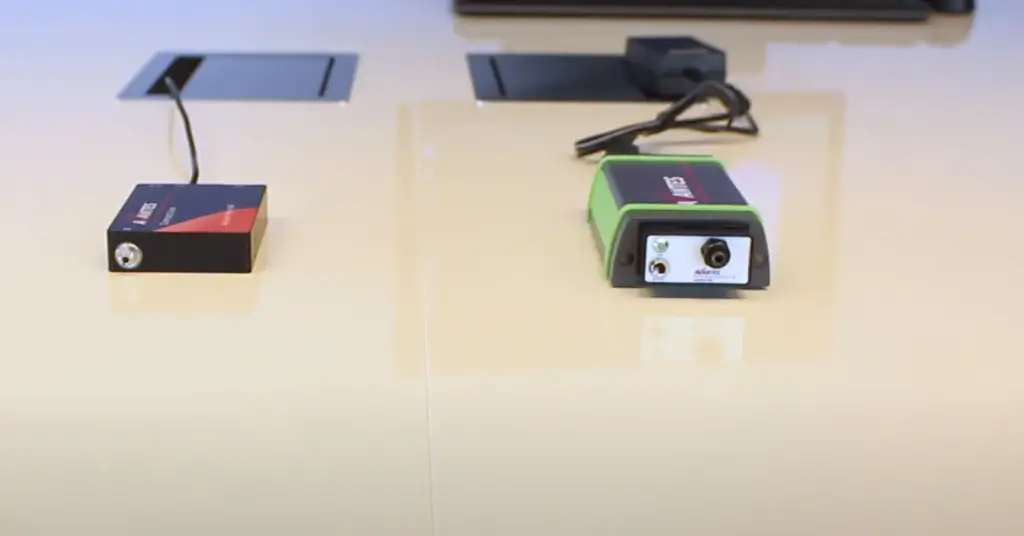## Use of Transmittance

Transmittance is a measure of how much light passes through a sample material.

It is often used in spectroscopic measurements and can be calculated using the formula: (I/Io) x 100%, where I is the amount of light that passes through the sample material, and Io is the amount of light that enters it. Transmittance can then be converted into absorbance by using the following equation: A = log10 (1/T), where T is transmittance expressed as a decimal fraction.

Absorbance values are useful for determining concentrations in spectrophotometric laboratory experiments because they follow Beer’s Law, which states that absorbance is directly proportional to concentration when all other variables remain constant. In other words, absorbance can be used to infer the concentration of a sample from its spectrophotometric reading.

The conversion from transmittance to absorbance is important in order to accurately interpret and compare spectrophotometer readings, which are typically given in terms of transmittance or absorbance. By understanding how to convert between the two, researchers can more easily analyze their results and draw meaningful conclusions about experiments that involve measuring light absorption through various materials. Ultimately, this increases confidence in laboratory research and allows for more accurate data collection and interpretation.

## Does High Transmittance Mean Low Absorbance?

No. Transmittance is the fraction of incident light that passes through a sample, and absorbance is the amount of energy absorbed by an object. The two measurements have an inverse relationship – a high transmittance means low absorbance, and vice versa. Therefore, it’s possible for a sample to have a high transmittance (i.e., to transmit more light) but still have high absorbance (i.e., absorb more energy).

## Can Transmittance Be Greater Than 100%?

No. Transmittance is a measurement of the fraction of incident light that passes through a sample, and therefore it can never be greater than 100%. If your transmittance reading is over 100%, then it’s likely an error in the measurement or a problem with the equipment.

If you wish to convert from transmittance to absorbance, there are two common methods for doing so: using Beer-Lambert’s Law or employing a calibration curve.

Beer-Lambert’s Law states that absorbance is proportional to the concentration of a substance and its path length. This means that if you know both of these parameters, you can calculate absorbance by dividing concentration by (path length x transmittance).## FAQ

### How do you convert transmittance to absorbance?

The formula for converting transmittance to absorbance is A = -log10(T/100), where T is the transmittance percentage and A is the absorbance. To calculate this, first one must convert the transmittance value from a percentage into a decimal by dividing it by 100. After that, take the logarithm of that decimal value with base 10; this will give you the absorbance value. For example, if you had a transmittance of 50%, then you would use 0.5 as your decimal value, which would yield an absorbance of 0.3 (A = -log10(0.5)). This conversion can be helpful when using spectrophotometers to measure light absorbance in various applications.

### Are there any other ways to convert transmittance to absorbance?

Yes, there are alternative methods for converting transmittance to absorbance such as the Lambert-Beer Law. This law states that the amount of light absorbed is proportional to the path length times the concentration of a chemical species. The formula for this law is A = εbc, where A is absorbance, ε is molar absorption coefficient (in Liters per mole), b is pathlength (in centimeters) and c is concentration (moles per Liter). Though this method doesn’t directly calculate from transmittance values, it can be used in tandem with them when analyzing samples with spectrophotometers. Additionally, some spectrophotometers come with their own software to convert transmittance values to absorbance values. This makes the process even simpler and more accurate than using manual calculations.

### What is the absorbance of 20% transmittance?

The absorbance of 20% transmittance is equal to 0.92. This can be calculated using the following equation: Absorbance = -log10(Transmittance/100). Therefore, for 20% transmittance, this calculation would look like this: Absorbance = -log10(20/100) = 0.92. The absorbance is a measure of how much of the light that passes through a material is absorbed by it. It is expressed on a logarithmic scale ranging from 0 (no absorption) to infinity (total absorption). Therefore, an absorbance of 0.92 indicates that 92% of the light was absorbed by the material and only 8% passed through.

### What absorbance corresponds to 50% transmittance?

To convert transmittance to absorbance, the formula A = log10 (1/T) is used. In this equation, T represents transmittance and A stands for absorbance. Therefore, when the transmittance is 50%, the corresponding absorbance can be calculated as follows: A = log10 (1/0.50) = 0.301029996 (= -log10(0.50)). This means that 50% transmittance corresponds to an absorbance of 0.301029996. It’s important to note that these equations only apply to ideal optical systems where there are no extraneous losses due to scattering or other effects. The accuracy of the results will depend on the conditions of the system under study. In conclusion, the equation A = log10 (1/T) enables the conversion of transmittance to absorbance. For example, 50% transmittance corresponds to an absorbance of 0.301029996. However, this equation only applies to ideal optical systems and its accuracy may vary depending on the conditions of the system under study.

### What is absorbance when transmittance is 100%?

When transmittance is 100%, absorbance is 0. This means that all of the light passing through the sample has been transmitted and none has been absorbed. The absorbance value of a sample with 100% transmittance is considered to be nonexistent because any amount of absorption would cause a decrease in transmittance. This value directly corresponds to the Beer-Lambert law, which states that for an infinitely thick sample, absorbance is equal to zero when transmittance equals one. In other words, if no light is being absorbed, then there could not possibly be any absorbance. To calculate absorbance from transmittance values less than 100%, the following formula can be used:Absorbance = -log10(Transmittance). This formula is used to convert from transmittance values to absorbance, and vice versa. It is important to note that this formula has its limitations; it only works for transmittance values between 0 and 1 (or 0% and 100%). For transmittance values outside of this range, a different equation must be used. Additionally, the Beer-Lambert law assumed that the sample being measured was infinitely thick. Thus, if the sample thickness changes or there are multiple layers present, the results may not accurately reflect the true absorbance value.

## Conclusion

In conclusion, the conversion of transmittance to absorbance is a straightforward process and can be done using either the Beer-Lambert Law or a more direct method. The Beer-Lambert Law uses a logarithmic scale to convert the transmitted light values into absorbance values, allowing scientists to accurately measure how much light is being absorbed by an object. Alternatively, the direct method takes advantage of the inverse relationship between transmission and absorption in order to calculate absorbance without having to use any logarithmic calculations. Whichever method you decide to use, it’s important that you understand how each works and are comfortable with your results. With this knowledge, you’ll be able to effectively monitor transmittance and absorbance in your samples.

Thanks for reading! We hope this article has been helpful in understanding how to convert transmittance to absorbance. Take the time to practice the steps and consider the different methods before you decide which one is best for your research. Good luck!